﻿ 变阻尼半主动振动控制算法减震效果计算研究 Computation Study on Algorithm for Structural Semi-Active Vibration Control with Variable Damps

Dynamical Systems and Control
Vol. 07  No. 04 ( 2018 ), Article ID: 26907 , 8 pages
10.12677/DSC.2018.74031

Computation Study on Algorithm for Structural Semi-Active Vibration Control with Variable Damps

Fangyuan Hou, Nan Ge*, Xinyuan Zhang

North China University of Science and Technology, Tangshan HebeiReceived: Aug. 30th, 2018; accepted: Sep. 14th, 2018; published: Sep. 21st, 2018ABSTRACT

A theoretical analyzing approach about vibration mitigation system, a 20 storey structure with variable dampers, was presented, and it was solved with software prepared with Matlab language. Results show that the system could have more notable vibration mitigation effect, which could still be optimized with reasonable increase of cdmax. A larger cdmax corresponds to larger seismic mitigation efficiency and larger semi-control forces. Seismic mitigation efficiency was generally 25% - 45%, with relatively even distribution along structural height. Semi-active control forces for both simple Bang-Bang and optimized Bang-Bang are approximately the same, with ones for optimized Bang-Bang slightly larger.

Keywords:State Variables, Riccati Equation, Principle Modes, Inter-Storey Drift, Runge-Kutta Method1. 引言

Hrovat  首先提出结构变阻尼半主动控制AVD系统，它实质上是一种节流孔的大小可以调节的粘性液体阻尼器，在每一采样周期内根据一定的控制律实时调节装置的阻尼特性，从而达到减震目的。该系统在美国、日本已有实际应用。Ruangrassamee等  提出粘滞–变摩擦阻尼算法，综合了粘滞阻尼器和摩擦阻尼器的优点。日本建成了Kajima Shizuoka变阻尼控制的建筑，并在实际小震作用下展示出了很好的控制效果  。孙作玉与李惠分别对建筑结构主动变阻尼控制进行了研究，并研制开发了主动变阻尼装置   。Yanqing Liu Hiroshi Matsuhisa  提出了新的半主动控制装置，由两个可变系数的阻尼器以及两个常数弹簧构成，给出了这种半主动控制装置的理论与试验分析结果。H. R. Owji，A. Hossain Nezhad Shirazi，H. Hooshmand Sarvestani  讨论了设有新型半主动调频质量阻尼器SADA-TMD以及主动调频质量阻尼器ATMD的结构反应特点。谭平，周福霖  基于变刚度和变阻尼的原理提出两种用于耦联结构体系的半主动控制装置，建立了这两种半主动控制耦联结构振动控制体系的运动方程，提出一种新的广泛适用于一般开关控制型半主动控制系统的控制算法。

2. 运动方程建立

2.1. 结构模型

2.2. 结构运动方程的建立

$M\stackrel{¨}{x}+C\stackrel{˙}{x}+Kx=F+{B}_{s}{U}_{s}$ (1)

$M=\left[\begin{array}{ccccc}{m}_{1}& 0& \cdots & & 0\\ {m}_{2}& {m}_{2}& 0& & 0\\ & & & & \\ ⋮& & & \cdots & ⋮\\ {m}_{n}& {m}_{n}& {m}_{n}& \cdots & {m}_{n}\end{array}\right]$ $C=\left[\begin{array}{ccccc}{c}_{1}& -{c}_{2}& \cdots & & 0\\ 0& {c}_{2}& -{c}_{3}& & 0\\ & & & & \\ ⋮& & & \cdots & -{c}_{n}\\ 0& 0& \cdots & 0& {c}_{n}\end{array}\right]$

$K=\left[\begin{array}{ccccc}{k}_{1}& -{k}_{2}& \cdots & & 0\\ 0& {k}_{2}& -{k}_{3}& & 0\\ & & & & \\ ⋮& & & \cdots & -{k}_{n}\\ 0& 0& \cdots & 0& {k}_{n}\end{array}\right]$ ${B}_{s}=\left[\begin{array}{ccccc}1& -1& \cdots & & 0\\ 0& 1& -1& & 0\\ & & & & \\ ⋮& & & \cdots & -1\\ 0& 0& \cdots & 0& 1\end{array}\right]$

${U}_{di}\left(t\right)=-{c}_{d}{\stackrel{˙}{x}}_{i}\left(t\right)$ (2)Figure 1. Structural modelFigure 2. Structural analysis model

1) 简单Bang-Bang变阻尼半主动控制算法可以表示为：

${U}_{di}\left(t\right)=\left\{\begin{array}{c}{c}_{d\mathrm{max}}\stackrel{˙}{x}\text{}\left[{x}_{i}\left(t\right)\stackrel{˙}{x}\left(t\right)>0\right]\\ {c}_{d\mathrm{min}}\stackrel{˙}{x}\text{}\left[{x}_{i}\left(t\right)\stackrel{˙}{x}\left(t\right)\le 0\right]\end{array}$ (3)

2) 最优Bang-Bang变阻尼半主动控制算法可以表示为：

${U}_{di}\left(t\right)=\left\{\begin{array}{c}{c}_{d\mathrm{max}}\stackrel{˙}{x}\text{}\left[{u}_{i}\left(t\right){\stackrel{˙}{x}}_{i}\left(t\right)<0\right]\\ {c}_{d\mathrm{min}}\stackrel{˙}{x}\text{}\left[{u}_{i}\left(t\right){\stackrel{˙}{x}}_{i}\left(t\right)\ge 0\right]\end{array}$ (4)

${c}_{d\mathrm{min}}$${c}_{d\mathrm{max}}$ 分别是主动变阻尼装置在伺服阀完全打开和关闭时的阻尼系数，也即装置能提供的最小阻尼系数和最大阻尼系数。ui体系最优控制力(可以是LQR最优控制或其他类型的最优控制) $U={\left[{u}_{1},{u}_{2},\cdots ,{u}_{n}\right]}^{T}$ 中楼层i的控制力。求最优控制力U的增益反馈矩阵 $P$ 的Ricatii方程为  ：

$PA+{A}^{T}P-PB{R}^{-1}{B}^{T}P+Q=0$

3. 计算实例与结果

4. 计算结果分析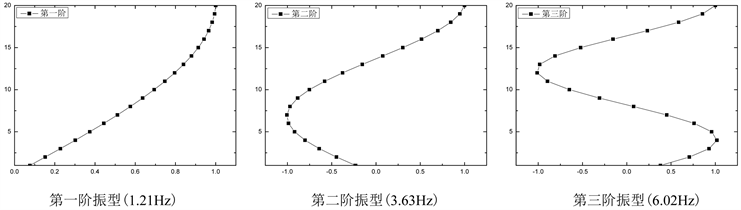Figure 3. Mode diagram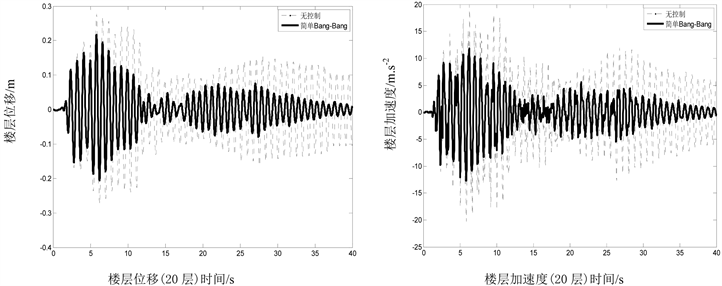Figure 4. Time histories of structural seismic dynamic response (simple Bang-Bang algorithm)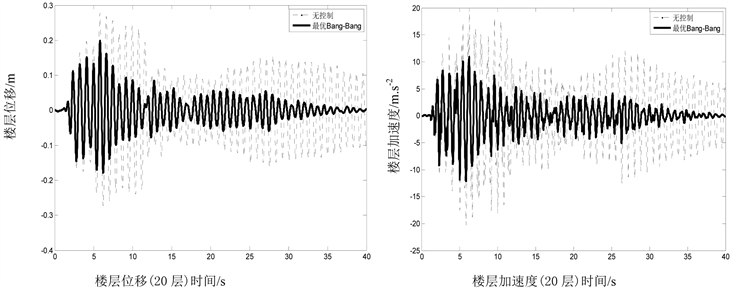Figure 5. Time histories of structural seismic dynamic response (optimal Bang-Bang algorithm)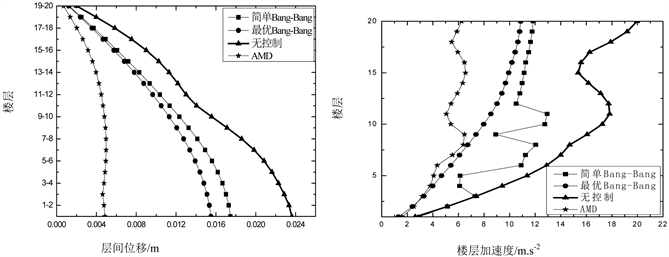Figure 6. Structural seismic dynamic response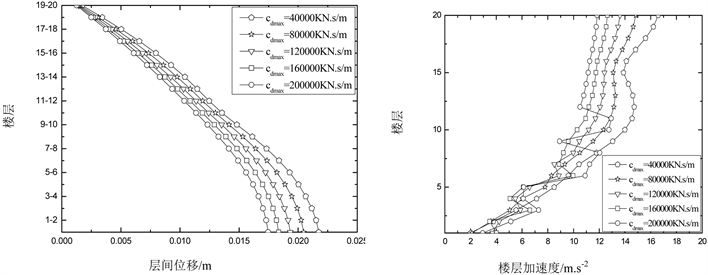Figure 7. Structural seismic dynamic response (simple Bang-Bang)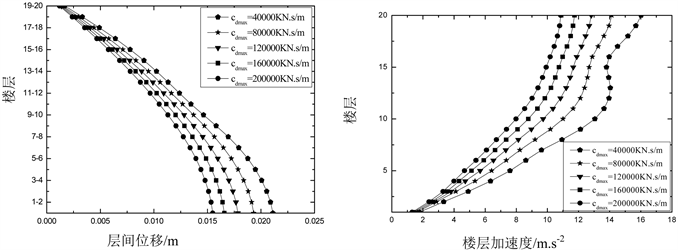Figure 8. Structural seismic dynamic response (optimal Bang-Bang)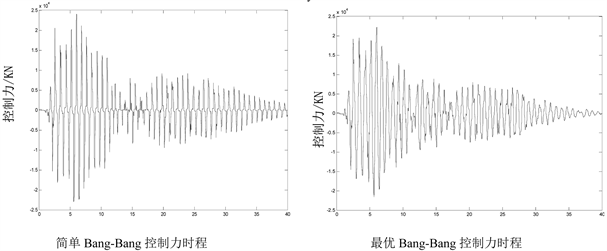Figure 9. Time histories for semi-active control forces

5. 结论

1) 变阻尼半主动控制对层间位移和楼层位移有较明显的减震效果，可以通过调整其参数指标cdmax达到目标减震效果，减震效率可达到25%~45%。

2) 简单Bang-Bang控制力与最优Bang-Bang基本相近，但最优Bang-Bang控制比简单Bang-Bang控制力稍大。cdmax越大，层间位移及楼层位移动力反应减震效率越高。

3) 变阻尼半主动控制装置对楼层加速度减震有一定效果。尤其是最优Bang-Bang效果比简单Bang-Bang好。

4) 本文所提供的数据和结论可以为多自由度半主动变阻尼系统在高层建筑的实验和实际应该用提供理论参考和对比。

Computation Study on Algorithm for Structural Semi-Active Vibration Control with Variable Damps[J]. 动力系统与控制, 2018, 07(04): 274-281. https://doi.org/10.12677/DSC.2018.74031

1. 1. Hrovat, D., Barak, P. and Rabins, M. (1983) Semi-Active versus Passive or Active Tuned Mass Dampers for Structural Control. Journal of Engineering Mechanics, 109, 691-705.
https://doi.org/10.1061/(ASCE)0733-9399(1983)109:3(691)

2. 2. Ruangrassamee, A., Sresamai, W. and Lukkunaprasit, P. (2006) Response Mitigation of the Base Isolated Benchmark Building by Semi-Active Control with the Viscous-Plus-Variable-Friction Damping Force Algorithm. Structural Control and Health Monitoring, 13, 809-822.
https://doi.org/10.1002/stc.113

3. 3. Kobori, T. (1998) Mission and Perspective towards Future Structural Control Research. Proceedings of the Second World Conference on Structural Control, 1, 25-34.

4. 4. 孙作玉, 隋丽丽. 变阻尼半主动结构振动台试验[J]. 地震工程与工程振动, 2000, 20(4): 106-111.

5. 5. 李惠, 袁雪松. 粘滞流体变阻尼半主动控制器对结构抗震控制的试验研究[J]. 振动工程学报, 2002, 15(1): 25-30.

6. 6. Liu, Y., Matsuhisa, H. and Utsuno, H. (2008) Semi-Active Vibration Isolation System with Variable Stiffness and Damping Control. Journal of Sound and Vibration, 313, 16-28.
https://doi.org/10.1016/j.jsv.2007.11.045

7. 7. Owji, H.R., Shirazi, A.H.N. and Sarvestani, H.H. (2011) A Comparison between a New Semi-Active Tuned Mass Damper and an Active Tuned Mass Damper. Procedia Engineering, 14, 2779-2787.
https://doi.org/10.1016/j.proeng.2011.07.350

8. 8. 谭平, 周福霖, 闫维明. 耦联结构体系的半主动控制[J]. 工业建筑, 2008, 38(7): 23-29.

9. 9. 欧进萍. 结构振动控制[M]. 北京: 科学出版社, 2003.

10. NOTES

*通讯作者。